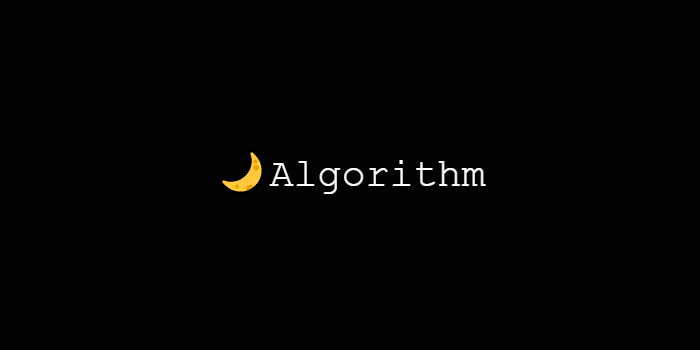# Leetcode :: Convert Sorted Array to Binary search Tree

wisepine·2021년 5월 25일
0

## 알고리즘

목록 보기
100/121### Problems

Given an integer array nums where the elements are sorted in ascending order, convert it to a height-balanced binary search tree.

A height-balanced binary tree is a binary tree in which the depth of the two subtrees of every node never differs by more than one.

### Guess

• 재귀로 두개의 subtree를 구성
• 반복적으로 root를 할당 (가운데값)

### Solution

# Definition for a binary tree node.
# class TreeNode:
#     def __init__(self, val=0, left=None, right=None):
#         self.val = val
#         self.left = left
#         self.right = right
class Solution:
def sortedArrayToBST(self, nums: List[int]) -> TreeNode:
if not nums:
return None

mid = len(nums) // 2

root = TreeNode(nums[mid])
root.left = self.sortedArrayToBST(nums[:mid])
root.right = self.sortedArrayToBST(nums[mid+1:])

return root